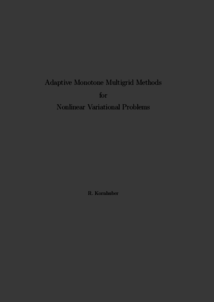Repository: Freie Universität Berlin, Math Department

# Adaptive monotone multigrid methods for nonlinear variational problems

Kornhuber, R. (1997) Adaptive monotone multigrid methods for nonlinear variational problems. Advances in Numerical Mathematics . Vieweg+Teubner Verlag, Stuttgart. ISBN 3519027224Preview

1MB

## Abstract

A wide range of problems occurring in engineering and industry is characterized by the presence of a free (i.e. a priori unknown) boundary where the underlying physical situation is changing in a discontinuous way. Mathematically, such phenomena can be often reformulated as variational inequalities or related non–smooth minimization problems. In these research notes, we will describe a new and promising way of constructing fast solvers for the corresponding discretized problems providing globally convergent iterative schemes with (asymptotic) multigrid convergence speed. The presentation covers physical modelling, existence and uniqueness results, finite element approximation and adaptive mesh–refinement based on a posteriori error estimation. The numerical properties of the resulting adaptive multilevel algorithm are illustrated by typical applications, such as semiconductor device simulation or continuous casting.

Item Type: Book Mathematical and Computer Sciences > Mathematics > Numerical Analysis Department of Mathematics and Computer Science > Institute of Mathematics 2000 Ekaterina Engel 02 Jan 2017 13:17 03 Mar 2017 14:42

Repository Staff Only: item control page